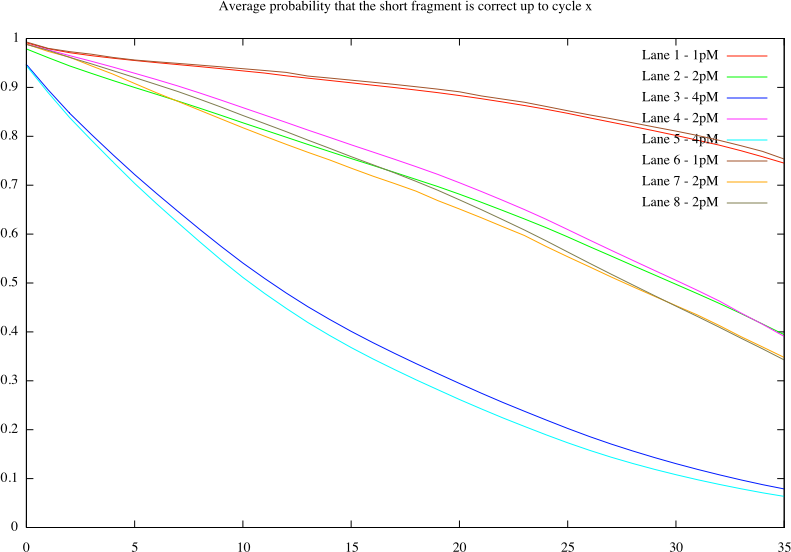Scientific

# Probability that a short fragment is correct

Below is a program we wrote to determine the probability of each short fragment based on the probaility scores as listed in the _prb files. The program has two modes of operation. In one it will print out the probability for each of the fragements that this short read is fully correct. In the second mode we can decide to shorten the fragments up to a certain probability.

To compile it type 'make probpersf' in the same directory where the program was downloaded. Below is a plot of the average probability up to cycle x. This plot is of course not enetirely correct since the number of clusters we obtain at a specific concentration is different for each of the lanes. As such these numbers should be treated as such. A better measure would probably be the median, but that has not yet been implemented.Mailing list - this is a general purpose mailing list for deep sequencing/sequencing by synthesis.